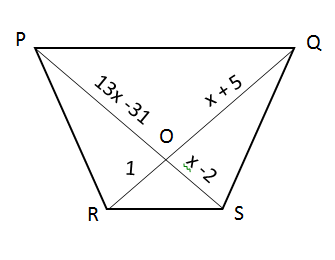# CAT Quantitative Aptitude Questions | Geometry Questions for CAT - Triangles

###### CAT Questions | CAT Geometry Questions | Quadrilaterals

The question is from CAT Geometry - Quadrilateral. Its a very basic question testing the basic properties of the quadrilaterals. CAT Geometry questions are heavily tested in CAT exam. Make sure you master Geometry problems.

Question 36: If PQ || RS, find the value of x1. 7
2. 3
3. Both A & B
4. None of these

## Best CAT Coaching in Chennai

#### CAT Coaching in Chennai - CAT 2021Online Batches Available Now!

##### Method of solving this CAT Question from Quadrilaterals: It is a very basic question.

If PQ || RS => ∆POQ ~ ∆ROS
=> $$frac{1}{$x+5$}$ = $\frac{x-2}{13x-31}\\$ => 13x - 31 =$x-2)(x+5)
=> 13x – 31 = x2 – 2x + 5x – 10
=> 13x – 31 = x2 + 3x – 10
=> x2 – 10x +21 = 0
=> x2 – 7x – 3x +21 = 0
=> x(x-7) -3(x-7) = 0
=> (x-3)(x-7) = 0
=> x = 3, 7

The question is "If PQ || RS, find the value of x "

##### Hence, the answer is Both A & B

Choice C is the correct answer.

###### CAT Coaching in ChennaiCAT 2021Enroll at 49,000/- 44,000/-

Online Classroom Batches Starting Now!

###### Best CAT Coaching in ChennaiPrices slashed by Rs 4000/-

Attend a Demo Class

## CAT Preparation Online | CAT Geometry questions Videos On YouTube

#### Other useful sources for Geometry Question | Geometry Triangles Circles Quadrilaterals Sample Questions

##### Where is 2IIM located?

2IIM Online CAT Coaching
A Fermat Education Initiative,
58/16, Indira Gandhi Street,
Kaveri Rangan Nagar, Saligramam, Chennai 600 093

##### How to reach 2IIM?

Phone: (91) 44 4505 8484
Mobile: (91) 99626 48484
WhatsApp: WhatsApp Now
Email: prep@2iim.com